# Olympiad Test: Problems Based On Figures - 2

## 20 Questions MCQ Test National Cyber Olympiad Class 8 | Olympiad Test: Problems Based On Figures - 2

Description
Attempt Olympiad Test: Problems Based On Figures - 2 | 20 questions in 40 minutes | Mock test for Class 8 preparation | Free important questions MCQ to study National Cyber Olympiad Class 8 for Class 8 Exam | Download free PDF with solutions
QUESTION: 1

Solution:
QUESTION: 2

Solution:
QUESTION: 3

### Select a suitable figure from the four alternatives that would complete the figure matrix.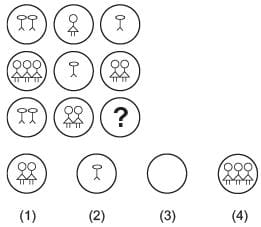Solution:
QUESTION: 4

Select a suitable figure from the four alternatives that would complete the figure matrix.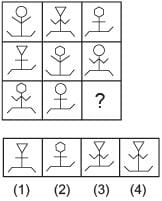Solution:
QUESTION: 5

Select a suitable figure from the four alternatives that would complete the figure matrix.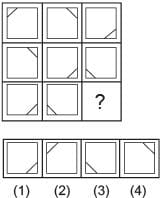Solution:
QUESTION: 6

Select a suitable figure from the four alternatives that would complete the figure matrix.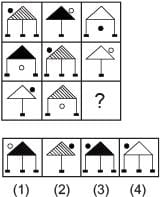Solution:
QUESTION: 7

Find out the alternative figure which contains figure (X) as its part.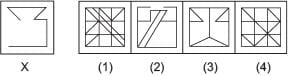Solution:
QUESTION: 8

Find out the alternative figure which contains figure (X) as its part.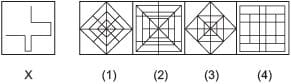Solution:
QUESTION: 9

Find out the alternative figure which contains figure (X) as its part.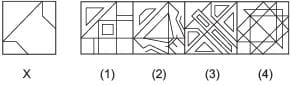Solution:
QUESTION: 10

Find out the alternative figure which contains figure (X) as its part.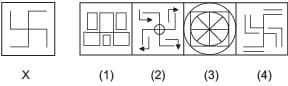Solution:
QUESTION: 11

Find out the alternative figure which contains figure (X) as its part.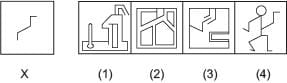Solution:
QUESTION: 12

Find out the alternative figure which contains figure (X) as its part.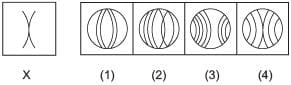Solution:
QUESTION: 13

Find out the alternative figure which contains figure (X) as its part.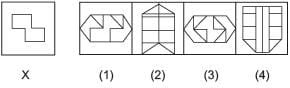Solution:
QUESTION: 14

Select a figure from amongst the Answer Figures which will continue the same series as established by the five Problem Figures.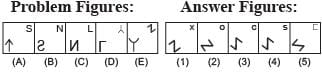Solution:
QUESTION: 15

Select a figure from amongst the Answer Figures which will continue the same series as established by the five Problem Figures.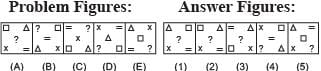Solution:
QUESTION: 16

Select a figure from amongst the Answer Figures which will continue the same series as established by the five Problem Figures.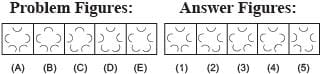Solution:
QUESTION: 17

Select a figure from amongst the Answer Figures which will continue the same series as established by the five Problem Figures.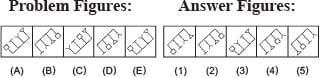Solution:
QUESTION: 18

Select a figure from amongst the Answer Figures which will continue the same series as established by the five Problem Figures.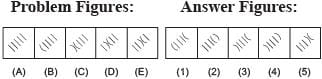Solution:
QUESTION: 19

Select the alternative which represents three out of the five alternative figures which when fitted into each other would form a complete square.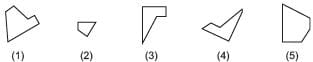Solution:
QUESTION: 20

Select the alternative which represents three out of the five alternative figures which when fitted into each other would form a complete square.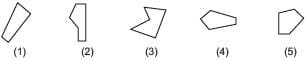Solution:Use Code STAYHOME200 and get INR 200 additional OFF Use Coupon Code